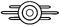40,570 PagesWelcome to the quiz for this lesson. Please enter your answers on your personal answer page.

##Namespace sectionProvide one example of a category, one example of a file, and one example of a main namespace article.

##Article layout sectionBelow is a list of article sections, but two are out of order. Which two sections should be flipped?

General section order
Navigation templates
Infobox
Lead section/introduction
Background
Characteristics
Locations
Appearances
Notes
Behind the scenes
Bugs
Gallery
See also
References
Navbox
Category

Vault Academy
Lessons Lesson one - Lesson two - Lesson three - Lesson four - Lesson five - Lesson six - Lesson seven - Lesson eight - Lesson nine - Lesson ten - Lesson eleven - Lesson twelve
Quizzes Quiz two - Quiz three - Quiz four - Quiz five - Quiz six - Quiz seven - Quiz eight - Quiz nine - Quiz ten - Quiz eleven - Quiz twelve

Links The Vault Academy - All lessons - Quiz answer report page
Community content is available under CC-BY-SA unless otherwise noted.